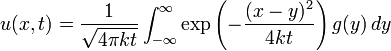# Heat Equation - Trouble Finding a General Solution

The_Chromer

Solve:

Ut=kUxx
U(x,0)=e^3x

## Homework Equations

The Heat Equation:## The Attempt at a Solution

g(y) in the heat equation for this problem is e^3y. I'm having serious trouble solving this because my professor hasn't taught us the method, and it isn't in the book. I've considered trying a change of variables by taking z=x-y, but this has led me to nowhere. I am lost, and I am not just fishing for a result.

I actually want to know how to solve this damn thing. There's a few pages worth of **** in my notebook, and now I need some guidance.

Homework Helper
Gold Member
The integral that you have to do is known as a Gaussian integral. A standard result, explained, for example, at http://en.wikipedia.org/wiki/Gaussian_integral is

$\int_{-\infty}^\infty \exp\left( - A u^2\right) du = \sqrt{\frac{\pi}{A}}.~~~(1)$

In your case, you must integrate

$\int_{-\infty}^\infty \exp\left( - \frac{(x-y)^2}{4kt} + 3y \right) dy.$

The technique needed is known as "completing the square," namely we attempt to write the quadratic expression in $y$ as a sum of a square plus a constant term:

$-A y^2 + B y + C = -a (y+b)^2 + c . ~~~(2)$

Here, $a,b,c$ will possibly be functions of $x,t,k$, but do not depend on $y$, so the resulting integral can be done by substituting $u = y +b$ and then using the formula (1).

You should probably start with equation (2) and determine $a,b,c$ in terms of $A, B,C$.

The_Chromer
I just wanted to let you know that I haven't given up on the problem and am still working at it.

I'll be back with results whenever I solve it.

The_Chromer
Thank you so much for your help. I reached the final solution. I'll upload the results to show you as soon as I can.

I have a final question as to what happened with a negative sign when I did a substitution, if you don't mind.